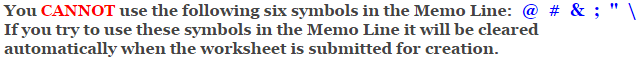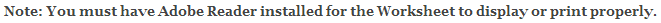# Algebraic Expressions Worksheets

## Evaluating One Variable Worksheets

This Evaluating One Variable Worksheet will create algebraic statements
for the student to evaluate.

You may select from 2, 3 and 4 terms with addition, subtraction, multiplication, and division.

### Type of Problems

 2 Terms (Addition and Subtraction) 3 Terms (Addition and Subtraction) 4 Terms (Addition and Subtraction) 3 Terms (Multiplication, Addition, and Subtraction) 4 Terms (Multiplication, Addition, and Subtraction) 2 Terms (Division, Addition, and Subtraction) 3 Terms (Division, Addition, and Subtraction)

### Type of Numbers to Use for the Variables

 Whole Numbers Integers

### Language for the Algebraic Expressions Worksheet

 English German Albanian Spanish Swedish Italian French Turkish Polish Norwegian

### Memo Line for the Algebraic Expressions Worksheet

You may enter a message or special instruction that will appear on the bottom left corner of the Algebraic Expressions Worksheet.### Algebraic Expressions Worksheet Answer Page

Now you are ready to create your Algebraic Expressions Worksheet by pressing the Create Button.

 Recommended Videos

If You Experience Display Problems with Your Math Worksheet